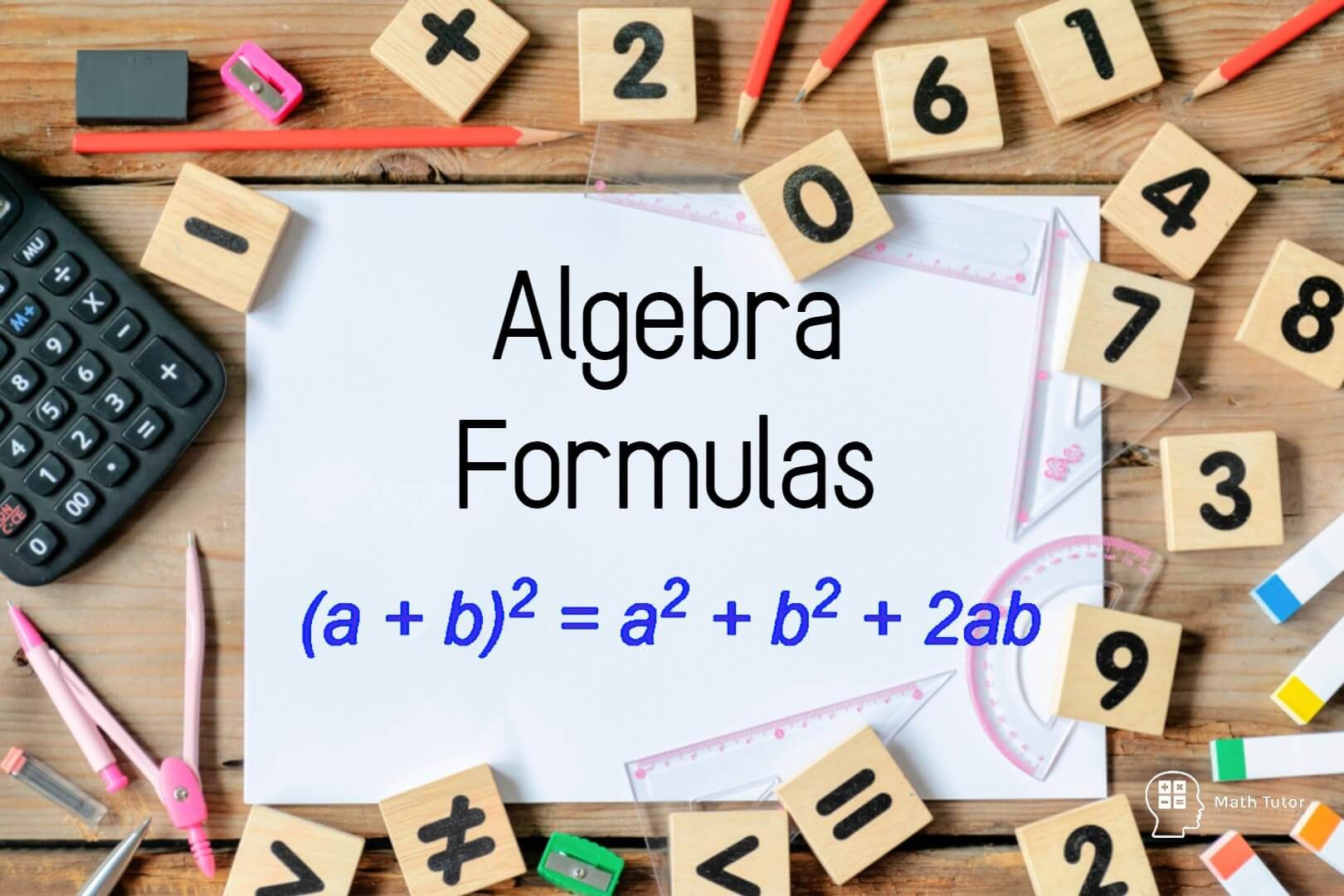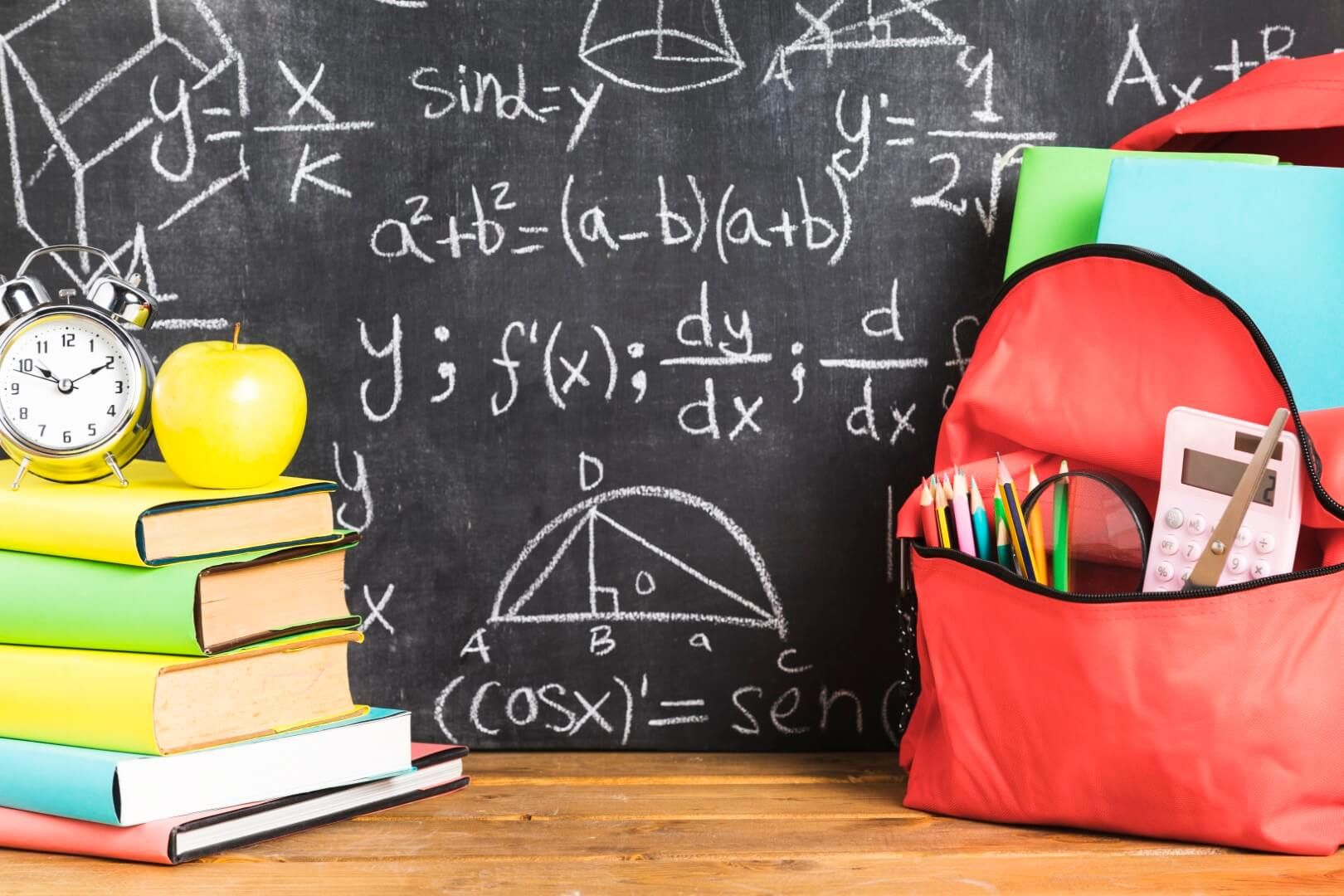## ALGEBRA## Algebra Formulas

In mathematics, Algebra is of the main parts of it. Algebra working with variables and numbers. Algebra is working with lots of formulas that relate two or more variables and numbers with each other. What is Formula? A relationship between several variables can be expressed as a formula. The value…## Algebra Basics

In this article, we are going to learn some algebra basics including, What are the algebraic symbols? Why do we use algebraic symbols? How to construct an algebraic expression for a given instance. At this moment, some of you may have an idea about algebra. Some may not. Let’s start…